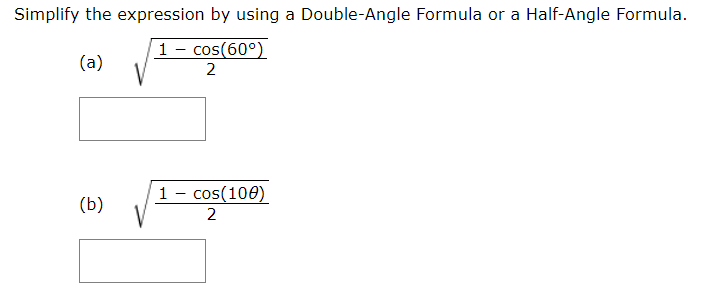# Simplify the expression by using a Double-Angle Formula or a Half-Angle Formula1- cos(60°)(a)21 - cos(100)(b)2

Question
2 viewshelp_outlineImage TranscriptioncloseSimplify the expression by using a Double-Angle Formula or a Half-Angle Formula 1- cos(60°) (a) 2 1 - cos(100) (b) 2 fullscreen
check_circle

Step 1

We need that half angle formula on the board.

Step 2

a) Theta = 60 , so theta/2 =30 degree

Using the f...

### Want to see the full answer?

See Solution

#### Want to see this answer and more?

Solutions are written by subject experts who are available 24/7. Questions are typically answered within 1 hour.*

See Solution
*Response times may vary by subject and question.
Tagged in

### Calculus